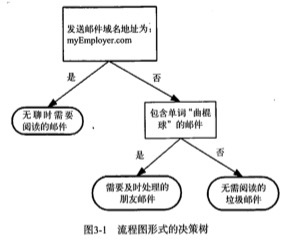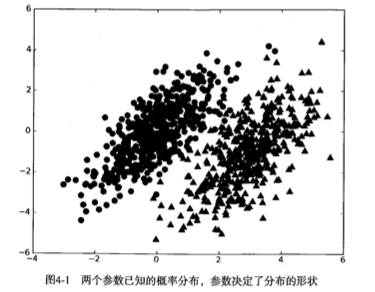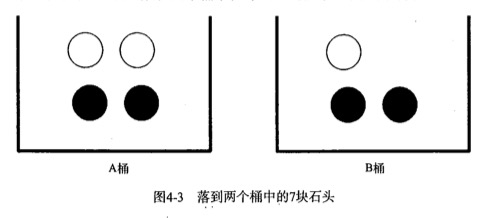构造

• 优点:在数据较少的情况下仍然有效，可以处理多类别问题;
• 缺点:对于输入数据的准备方式较为敏感;
• 使用数据类型:标称型。

流程

1. 收集数据:可以使用任何方法;
2. 准备数据:需要数值型或者布尔型数据;
3. 分析数据:有大量特征时，绘制特征作用不大，此时使用直方图效果更好;
4. 训练算法:计算不同的独立特征的条件概率;
5. 测试算法:计算错误率;
6. 使用算法:一个常见的朴素贝叶斯应用是分档分类。可以在任意的分类场景中使用朴素贝叶斯分类器，不一定非要是文本。

理论

贝叶斯决策• 如果p1(x, y) > p2(x, y)，那么类别为1;
• 如果p2(x, y) > p1(x, y)，那么类别为2。

条件概率$$P(gray|bucketB) = P(gray\ and\ bucketB) / P(bucketB)$$

$$P(c|x) = \frac{P(x|c)P(c)}{P(x)}$$